# Reversal process in DFA

Prerequisite – Designing finite automata
Reversal : Reversing a language means reversing the each string in the language.

Steps to Reversal:

1. Draw the states as it is.
2. Make final state as initial state and initial states as a final state
3. Reverse the edges
4. Loop will remain same
5. Remove the inappropriate transition state.

Note :
1. Not every reversal of DFA lead to DFA
2. Reversal process goes like this: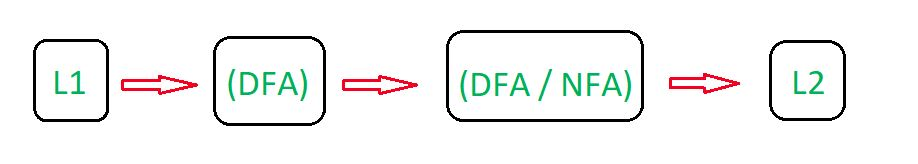Let’s understand the Reversal process in Deterministic Finite Automata (DFA) with the help of below example.

Example-1:
Designing a DFA for the set of string over {a, b} such that string of the language start symbol ‘a’. The desired language will be formed:

L1 = {a, aa, ab, aab, aaabb, aabab, .......}

In L1, each string have starting element a. State Transition Diagram for the language L1: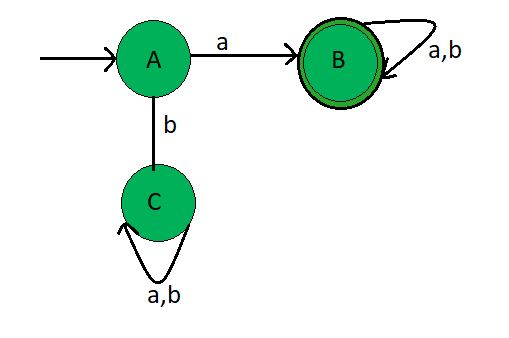This DFA accepts all the string starting with a. Here, State A and C are normal state but state B is final state. Now, Reversing the L1 language which gives the final result of the language L2.

State Transition Diagram of L2 (reverse of L1):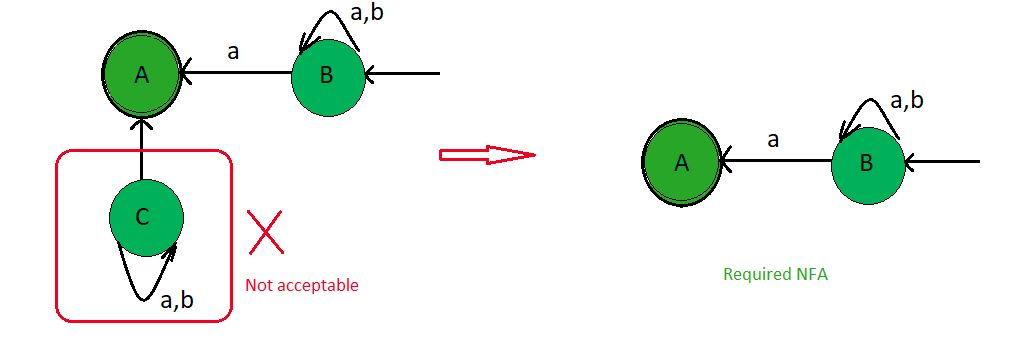Thus as we see that L2 have been obtained through reversal process and this final NFA accept all the language containing strings ending with a symbol ‘a’.

l = {a, ba, bba, ababa, ......}

Explanation:

• Same states (A, B, C) are draw as present in original state diagram
• Since A is the initial state make it as final state.
• Since B is the final state make it as initial state.
• Reversing the edges, since previously edges point A to B and A to C .Change the direction, now edges points toward B to A and C to A.
• Assign the values as same as original.
• Draw the loop as in original state diagram
• Since there is no incident edge on the state C so, we can reduce this state C.
• Since there is no transition for ‘a’ and ‘b’ in state A .Therefore resultant FA is NFA.

Example-2:
Designing a DFA for the set of string over {a, b} such that string of the language contain even number of’a’. The desired language will be formed:

L1 = {, aa, aab, aba, aab, aababa, .......}

In L1, each string having even number of element a.

State Transition Diagram for the language L1: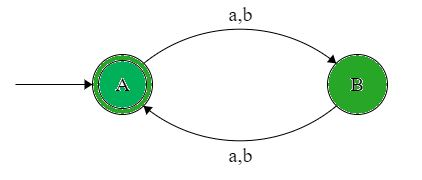This DFA accepts all the string containing even number of element a.Here, State A is initial state and state B is final state. Now, Reversing the L1 language which gives the final result of the language L2.

State Transition Diagram of L2 (reverse of L1):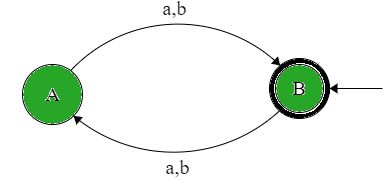Thus as we see that L2 have been obtained through reversal process and this final DFA accepts all the string containing even number of element a which is same as the original language.

L2 = {, aa, aab, aba, aab, aababa, .......}

Explanation:

• Same states (A, B) are draw as present in original state diagram.
• Since A is the initial state make it as final state.
• Since B is the final state make it as initial state.
• Reversing the edges, since previously edges point A to B and so on.Change the direction, now edges points toward B to A and so on.
• Assign the values as same as original.
• Since there is transition for ‘a’ and ‘b’ in state A and B.Therefore resultant FA is DFA.
• Since State diagram after and before reversal are same.Therefore L1 = L2My Personal Notes arrow_drop_upSmall things always make you to think big

If you like GeeksforGeeks and would like to contribute, you can also write an article using contribute.geeksforgeeks.org or mail your article to contribute@geeksforgeeks.org. See your article appearing on the GeeksforGeeks main page and help other Geeks.

Please Improve this article if you find anything incorrect by clicking on the "Improve Article" button below.